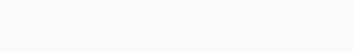## Incremental analysis

• boost，抬升电压的大小为$$V_D$$，抬升后，LED两端的电压围绕$$V_D$$为中心，上下对称波动；
• shrink，即减少正弦波的振幅，也就是将正弦波的波峰和波谷压缩到正好对应于所选取的LED 特性曲线中那一小段线性区所对应的的最大电压和最小电压；takeaway  要点

## Dependent Sources and Amplifiers

• Nonlinear circuits — can use the node method；
• Small signal trick resulted in linear response；

### Amplifiers

amplifier放大器的要点：

• Signal amplification key to both analog and digital processing.
• Besides the obvious advantages of being heard farther away, amplification is key to noise tolerance during communcation.
• 模拟信号向数字信号转换需要信号的强度是一个特定值，这样才能转换成数字电平，所以如果原始模拟信号很小，就要利用放大器放大。
• 通常放大器除了有输入和输出，还可能包含电源端口(power port)，因为放大的信号意味着通过放大器实现信号功率的增益。• We often don’t show the power port.
• Also, for convenience we commonly observe "the common ground discipline". In other words, all ports often share a common reference point called "ground".### Dependent Source

dependent source是一种新的device，如果看作黑箱的话，那么有2个-port，control port和output port，两个port分别有正负两个接线端，如图所示。可以有多种形式：

• Voltage Controlled Current Source ：简写为VCCS，也就是Current at output port is a function of voltage at the input port；
• Current Controlled Voltage Source
• Voltage Controlled Voltage Source
• Current Controlled Current Sourcewhere's the amplification? 我们给定一些参数之后，就可以得到上面蓝色框中更具体的表达式了。• If VCCS is a device that can source power(就是说VCCS向外供电), then the mathematically predicted behavior will be observed(也就是上图的曲线可以降到零，然后继续降为负值).
• 如果VCCS是一个【passive device】，那么它就不能source power，于是物理上$$v_o$$不可能为负值。那么不断增大$$v_I$$，使得$$v_o$$靠近零，此时会发生什么呢？难道是按照上面的数学公式，可以无限逼近上图的交点，只是不能取到吗？## MOSFET amplifier large signal analysis

• Amp constructed using dependent source• Dependent source in a circuit• Superposition with dependent sources: one way → leave all dependent sources in; solve for one independent source at a time [section 3.5.1 of the text]不要去管受控源，仅仅开关你的独立源就可以了，然后观察单独开始一个独立源下的响应，最后把所有响应叠加，整个过程中受控源都保留在电路中。
• 放大器Switch Current Source Model(SCS model)
When $$v_{G S} \geq V_T$$, and also $$v_{D S} \geq v_{G S}-V_T$$, the MOSFET is in its Saturation Region, and the SCS model of the MOSFET is more accurate than the S or SR model. 此时MOSFET被揍扁了，崩溃了，放弃抵抗躺平了(即$$i_{D S}$$不再随着$$v_{D S}$$的增长而增值，而是保持不变，注意在SR model这两者是线性关系)，MOSEFT就会在drain and source之间表现出电流源的特性，所以我们用SCS model MOSFET as a current source in that part。

• S model— just for fun!
• SR model—在整个数字电路design中，我们关注SR model。比如一个下拉的MOSFET管开启的时候，或者许多MOSFET管开启的时候，输出电压就被拉到接近于地，所以$$v_{D S}$$很小，应用SR model make sense. ；
• SCS model—在模拟电路，比如放大器设计或者类似情况，we will be operating the MOSFET in a a saturation regeion。当讨论模拟电路的放大器的时候，我要求你们遵守这个规则，即饱和规则，就是说将工作在饱和区的MOSFET管作为一个电流源。

Note: alternatively (in more advanced courses)
$$v_{D S} \geq v_{G S}-V_T$$   use SCS model
$$v_{D S}<v_{G S}-V_T$$   use SR model
or, use SU Model (Section 7.8 of A&L)  也可以参考这里：Switch Unified (SU) MOSFET ModelLet's analyze the circuit. First, replace the MOSFET with its SCS model. 那么在给定电路元件和连接方式，以及control port (GS端)的电压的情况下，$$v_O$$以及$$i_{D S}$$是多少呢？下面用图像法形象展示求解方法。## Amplifiers - small signal model对于上面讨论的MOSFET，我们会碰到之前在学习放大器的时候遇到过的问题，即放大过程会导致放大后信号的distortion，即不能实现线性放大。这里我们采用的方法同样是禅宗，即只专注输入-输出电压关系式曲线上的一小段。Good choice for operating point: midpoint of input operating range. 即上图红色圈出的。How to choose the bias point:

• gain component—这里是指$$g_m=K\left(V_I-V_T\right)$$被称作跨导(transconductance)，叫这个名字是因为它在某种程度上反映了对输入的传导能力。
• gain—$$A=-K\left(V_I-V_T\right) R_L$$
• 如果偏置点选择不当，当信号变大时可能被截断或者产生失真distortion
• Input valid operating range. Bias at midpoint of input operating range for maximum swing.  比如你想在正半周和负半周获得同样的偏移。xxx

in glory

glitch

precipitously

shattering

wimp

grubby

articulation

embellish

in series with 串联 （The resistor, which is in series with the capacitor）